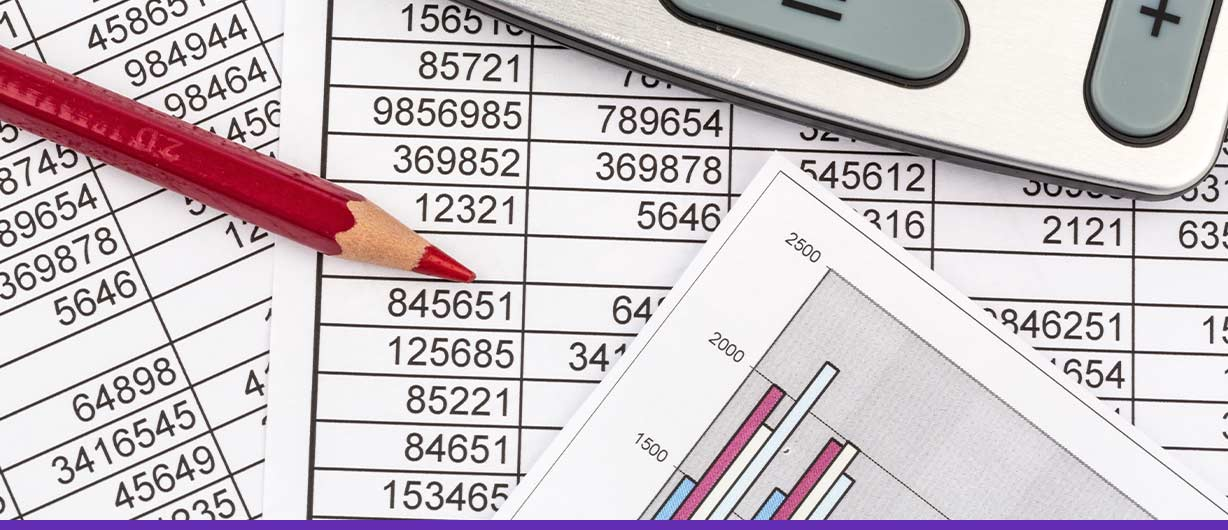# What Is Straight Line Method Of Depreciation?

Farwah Jafri | November 3 2022

Depreciation is a process that defines the gradual decrease of the cost of a fixed asset over a period of time while it is in use.

Depreciation plays a vital role in the accounting process of the business as it allows the company to recognize the devaluation of the asset over time. This helps in getting a more realistic picture of profitability.

In simpler words, depreciation is more about cost allocation than asset valuation. Depreciation largely impacts the financial statements of a company. Statements like the income statement and the balance sheet; therefore, it is very crucial to get it right.

There are many ways by which depreciation is calculated which comply with GAAP (Generally Accepted Accounting Principles).The most commonly used method to calculate depreciation is the straight line method.

## Straight Line Method of Depreciation

The straight line method is the most straightforward way of calculating the value of an asset over its useful life.

According to Investopedia straight line method is “a method of accounting for depreciation that assumes an asset loses value at a constant rate.”

The straight line method uses only three variables to calculate the depreciation value.

Fixed assets like property, equipment, or machinery are expected to last more than a year. They are expensive items. Depreciation is supposed to smooth out their purchasing costs over the time they are useful.

Straight line depreciation method depends upon the cost of an asset over time which is known as “useful life. It can be calculated with the help of the cost of the asset and useful life and an estimated salvage value.

A salvage value means how much the asset is likely to be worth of by the end of its useful life.

In a straight line method formula, the depreciation value remains uniform, while the book value of the asset reaches its salvage value.

## Straight Line Method Formula

The straight line depreciation formula is:

Annual Depreciation Expense = (Cost Of The Asset – Salvage Value) /Useful Life Of The Asset

Here:

o  The cost of the asset is the actual price of the asset.

o  Salvage value is the value of the asset which will remain at the end of its useful life.

o  The useful life of the asset is the life expectancy of the asset represented in a number of years.

## Merits And Demerits Of The Straight Line Method of Depreciation

### Merits Of Straight Line Method

The merits of a straight line depreciation method are:

o  It is easy to calculate because of the consistency of the depreciation amount.

o  It can easily apply to different fixed assets, especially when they tend to diminish over the passage of time.

o  The schedules are easy-to-understand and simple.

### Demerits Of The Straight Line Method

The demerits of this depreciation calculation method are:

o  Some assets depreciate differently depending upon their usage, input, or output. It becomes difficult to calculate the correct depreciation rate if the straight line method is used.

o  Some assets experience accelerated salvation in their early years. Assets like vehicles and computers; thus, this method doesn’t give an accurate result.

o  The estimates for salvage value and useful life can be inconsistent and subjective in different companies.

Accountants generally favor the straight line depreciation method as it is very easy to use and renders fewer errors over the useful life of an asset with regard to its expenses at the same amount every year.

There are other methods to calculate depreciation, but they are complex, such as the double declining balance method.

For instance, there is a risk that technical advancements can potentially render an asset which becomes obsolete earlier than projected. The straight line method will not be able to calculate the accurate depreciation amount. As such a short useful life of the asset nor the extra cost needed for its maintenance to keep it working.

This can cause additional burden to the company, which may not be considered in straight line method of depreciation.

## When Should The Straight Line Method Be Used?

It is best to use a straight line method for depreciation if it is known that the value of the asset will decrease at a steady rate over time. There are fixed items like machinery etc. They depreciate at a steady pace, and the depreciation remains almost uniform throughout their useful life.

## Final Thoughts

Straight line depreciation method is a popular way of allocating the cost of fixed assets over their useful life duration. This is the most common method in practice and is in compliance with GAAP, despite having its own set of drawbacks.## Author Bio

Farwah is the Product Owner of Monily. She has an MBA from Alliance Manchester Business School, UK. She is passionate about helping businesses overcome challenges that hamper their growth, which is why she is working at Monily to facilitate entrepreneurs to efficiently manage business finances and stay focused on growth.